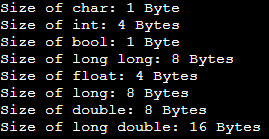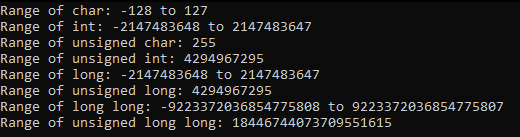# Size and Range of Data Types in C++

## Size and Range of Data Types in C++:

In this article, I am going to discuss the Size and Range of Data Types in C++ with Examples. Please read our previous article where we discussed Data Types in C++ with Examples.

##### Size and Range of Data Types in C++:

Let us look at the data types and their sizes. We can print the size of any data type with the help of sizeof() operator. So, we have a program that will print the size of all the data types.

##### Program to Show the Size of Data Types in C++:
```#include <iostream>
using namespace std;
int main()
{
cout<< "Size of char: " << sizeof(char) << " Byte" << endl;
cout<< "Size of int: " << sizeof(int) << " Bytes" << endl;
cout<< "Size of bool: " << sizeof(bool) << " Byte" << endl;
cout<< "Size of long long: " << sizeof(long long) << " Bytes" << endl;
cout<< "Size of float: " << sizeof(float) << " Bytes" << endl;
cout<< "Size of long: " << sizeof(long) << " Bytes" << endl;
cout<< "Size of double: " << sizeof(double) << " Bytes" << endl;
cout<< "Size of long double: " << sizeof(long double) << " Bytes" << endl;
return 0;
}
```
###### Output:So, these are the sizes of some important data types. Now let us print the range of these data types.

##### Program to Show the Range of Data Types in C++:
```#include <iostream>
using namespace std;
int main()
{
cout<< "Range of char: " << CHAR_MIN  << " to " << CHAR_MAX<< endl;
cout<< "Range of int: " << INT_MIN  << " to " << INT_MAX<< endl;
cout<< "Range of unsigned char: " << UCHAR_MAX << endl;
cout<< "Range of unsigned int: " << UINT_MAX << endl;
cout<< "Range of long: " << LONG_MIN  << " to " << LONG_MAX << endl;
cout<< "Range of unsigned long: " << ULONG_MAX << endl;
cout<< "Range of long long: " << LONG_LONG_MIN  << " to " << LONG_LONG_MAX << endl;
cout<< "Range of unsigned long long: " << ULONG_LONG_MAX << endl;

return 0;
}
```
###### Output:So, these are the range of signed and unsigned data types.